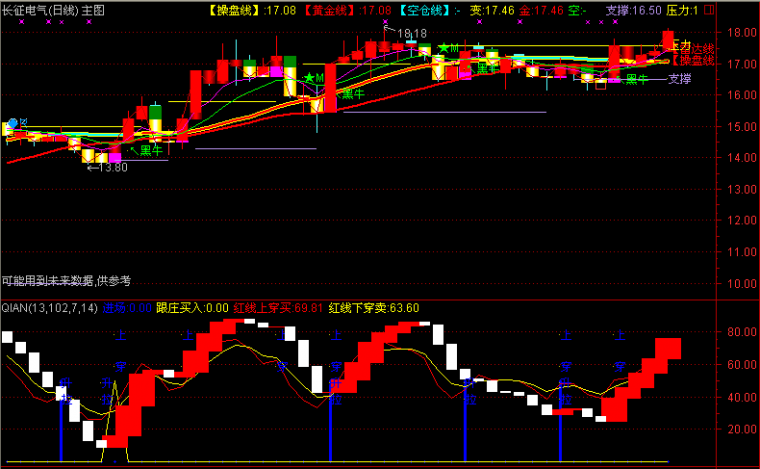﻿ 找准起爆点指标公式源码 有图有真相-通达信公式 －程序化交易（CXH99.COM）

# 找准起爆点指标公式源码 有图有真相[通达信公式]

N赋值:99
N1赋值:99
M1赋值:3
M2赋值:1
WR1赋值:100*(N日内最高价的最高值-收盘价)/(N日内最高价的最高值-N日内最低价的最低值)
WR2赋值:100*(N1日内最高价的最高值-收盘价)/(N1日内最高价的最高值-N1日内最低价的最低值),画洋红色
RSV赋值:(收盘价-N日内最低价的最低值)/(N日内最高价的最高值-N日内最低价的最低值)*100
K赋值:RSV的M1日[1日权重]移动平均
D赋值:K的M2日[1日权重]移动平均
J赋值:3*K-2*D
K上穿WR2

N:=99;
N1:=99;
M1:=3;
M2:=1;
WR1:=100*(HHV(HIGH,N)-CLOSE)/(HHV(HIGH,N)-LLV(LOW,N));
WR2:=100*(HHV(HIGH,N1)-CLOSE)/(HHV(HIGH,N1)-LLV(LOW,N1)),COLORMAGENTA;
RSV:=(CLOSE-LLV(LOW,N))/(HHV(HIGH,N)-LLV(LOW,N))*100;
K:=SMA(RSV,M1,1);
D:=SMA(K,M2,1);
J:=3*K-2*D;
CROSS(K,WR2);

{程序化交易 www.cxh99.com }
{程序化交易 www.cxh99.com }(注：由于人数限制，QQ或微信请选择方便的一个联系我们就行，加好友时请简单备注下您的需求，否则无法通过。谢谢您！)

【字体： 】【打印文章】【查看评论

没有相关内容